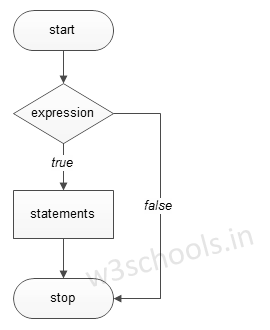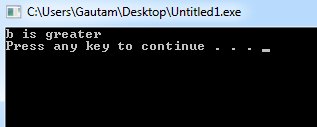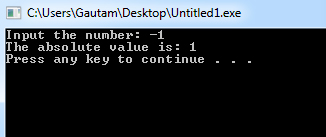##### C++ Programming Tutorial Index

If statements in C++ is used to control the program flow based on some condition, it's used to execute some statement code block if the expression is evaluated to true. Otherwise, it will get skipped. This is the simplest way to modify the control flow of the program.

The if statement in C++ can be used in various forms depending on the situation and complexity.

There are four different types of if statement in C++. These are:
• Simple if Statement
• if-else Statement
• Nested if-else Statement

The basic format of if statement is:

Syntax:
``````if(test_expression)
{
statement 1;
statement 2;
...
}``````

'Statement n' can be a statement or a set of statements, and if the test expression is evaluated to true, the statement block will get executed, or it will get skipped.

Figure - Flowchart of if Statement:

###Example of a C++ Program to Demonstrate if Statement

Example:
``````#include <iostream>
using namespace std;

int main()
{
int a = 15, b = 20;

if (b > a) {
cout << "b is greater" << endl;
}
system("PAUSE");
}``````
Program Output:Example:
``````#include <iostream>
using namespace std;

int main()
{
int number;

cout << "Input the number: ";  cin >> number;

/* check whether the number is negative number */
if(number < 0)
{
/* If it is a negative then convert it into positive. */
number = -number;
cout<<"The absolute value is: "<< number<<endl;
system("PAUSE");
}
} ``````
Program Output: